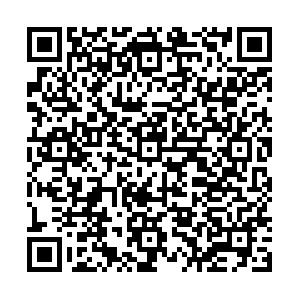EI、Scopus 收录

## 留言板引用本文: 陈婷婷, 王凯, 成利, 周加喜. 准零刚度驱动式压电低频振动能量采集方法. 力学学报, 2023, 55(10): 1-12Chen Tingting, Wang Kai, Cheng Li, Zhou Jiaxi. Research on quasi-zero-stiffness-enabled piezoelectric low-frequency vibration energy harvesting method. Chinese Journal of Theoretical and Applied Mechanics, 2023, 55(10): 1-12 doi: 10.6052/0459-1879-23-315
 Citation: Chen Tingting, Wang Kai, Cheng Li, Zhou Jiaxi. Research on quasi-zero-stiffness-enabled piezoelectric low-frequency vibration energy harvesting method. Chinese Journal of Theoretical and Applied Mechanics, 2023, 55(10): 1-12• 中图分类号: O313

## RESEARCH ON QUASI-ZERO-STIFFNESS-ENABLED PIEZOELECTRIC LOW-FREQUENCY VIBRATION ENERGY HARVESTING METHOD

• 摘要: 如何高效且经济环保地为数以万计的传感器网络节点供电, 是物联网快速发展和大范围应用的瓶颈性难题. 将振动能转换为电能以实现传感器自供电是物联网传感器网络节点供能的潜在方案. 但是, 环境振动中低频成分占比较大, 而传统振动能量采集方法较难实现低频( < 10Hz)振动能量的高效转化, 这限制了振动能量采集技术在物联网领域的大范围应用. 文章提出了一种准零刚度驱动式压电振动能量采集装置, 可将环境、人体及部分机械设备的低频振动能量高效地转化为电能. 首先利用能量法得到压电俘能单元的机电耦合方程, 并用谐波平衡法获得系统动力学及电学响应的解析表达式, 同时对比了数值解与解析表达式的结果; 进一步探究了阻尼比、激励幅值等参数对动力学响应及电学输出的影响. 最后加工制备了准零刚度驱动式压电振动能量采集装置样机, 搭建了实验平台, 测试了系统的动力学响应与电学输出, 验证了理论结果的正确性. 研究结果显示当频率为2.5Hz时, 准零刚度驱动式压电振动能量采集装置中单个能量转化单元的最大峰值电压达到25V. 文章提出的准零刚度压电能量采集装置有望克服传统共振型压电能量采集装置能量采集频带依赖于能量采集系统固有频率以及多稳态能量采集器需要跨越势垒而难以实现超低频小幅值能量俘获的难题, 进一步夯实振动能量采集理论, 为超低频小振幅振动能量的高效采集提供新思路.

• 图  1  俘能装置结构模型图

Figure  1.  Structure model diagram of energy harvesting device

图  2  复合梁模型及其截面图

Figure  2.  Composite beam model and its cross section

图  3  回复力和机电耦合系数的原函数和拟合函数图($\gamma = 1.56{\text{25}}$, $\theta = 0.21$,$A = 0.17$,$B = 0.{\text{25}}$)

Figure  3.  The original function and polynomial approximation diagram of restoring force and electromechanical coupling coefficient ($\gamma = 1.56{\text{25}}$, $\theta = 0.21$,$A = 0.17$,$B = 0.{\text{25}}$)

图  4  数值结果与解析解结果对比($\gamma = 1.56{\text{2 5}}$, $\zeta = 0.055$, $\rho = 2$,$\theta = 0.21$, u0 = 0.03)

Figure  4.  Comparison of numerical results and analytical results ($\gamma = 1.56{\text{2 5}}$,$\zeta = 0.055$, $\rho = 2$,$\theta = 0.21$, u0 = 0.03)

图  5  阻尼对位移及电压响应的影响($\gamma = 1.56{\text{2 5}}$,$\rho = 2$,$\theta = 0.21$, u0 = 0.03)

Figure  5.  Influence of damping on displacement and voltage response ($\gamma = 1.56{\text{2 5}}$,$\rho = 2$,$\theta = 0.21$, u0 = 0.03)

图  6  激励幅值对位移和输出电压响应的影响($\gamma = 1.56{\text{2 5}}$,$\zeta = 0.055$, $\rho = 2$,$\theta = 0.21$)

Figure  6.  Influence of excitation amplitude on displacement and output voltage($\gamma = 1.56{\text{2 5}}$,$\zeta = 0.055$, $\rho = 2$,$\theta = 0.21$)

图  7  实验设备装置图

Figure  7.  Picture of experiment setup

图  8  不同激励幅值下绝对位移响应与峰值电压的输出结果

Figure  8.  Output results of absolute displacement response and peak voltage under different excitation amplitudes

图  9  在2.5 Hz时, 不同激励幅值的位移时域响应曲线

Figure  9.  At 2.5 Hz, the displacement time-domain response curve with different excitation amplitude

表  1  模型的相关物理参数

Table  1.   Relevant parameters of the prototype

 Item Symbol Value thickness of the beam bp 10 mm thickness of the film hp 0.1 mm weight of proof mass m 200 g elastic moduli of the beam Ys 196 GPa elastic moduli of the film Yp 3 GPa permittivity constant of the film h33 106 mF/m piezoelectric stress constant e31 65 Mc/m2
•点击查看大图
##### 计量
• 文章访问数:  131
• HTML全文浏览量:  41
• PDF下载量:  47
• 被引次数: 0
##### 出版历程
• 网络出版日期:  2023-08-16

### 目录/下载:  全尺寸图片 幻灯片
• 分享
• 用微信扫码二维码

分享至好友和朋友圈